# Recent Activity

## Arc-disjoint out-branching and in-branching ★★

Author(s): Thomassen

Conjecture   There exists an integersuch that every-arc-strong digraphwith specified verticesandcontains an out-branching rooted atand an in-branching rooted atwhich are arc-disjoint.

Keywords:

## Strong edge colouring conjecture ★★

Author(s): Erdos; Nesetril

A strong edge-colouring of a graphis a edge-colouring in which every colour class is an induced matching; that is, any two vertices belonging to distinct edges with the same colour are not adjacent. The strong chromatic index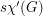is the minimum number of colours in a strong edge-colouring of.

ConjectureKeywords:

## Long directed cycles in diregular digraphs ★★★

Author(s): Jackson

Conjecture   Every strong oriented graph in which each vertex has indegree and outdegree at leastcontains a directed cycle of length at least.

Keywords:

## Splitting a digraph with minimum outdegree constraints ★★★

Author(s): Alon

Problem   Is there a minimum integer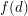such that the vertices of any digraph with minimum outdegreecan be partitioned into two classes so that the minimum outdegree of the subgraph induced by each class is at least?

Keywords:

## Stable set meeting all longest directed paths. ★★

Author(s): Laborde; Payan; Xuong N.H.

Conjecture   Every digraph has a stable set meeting all longest directed paths

Keywords:

## Ádám's Conjecture ★★★

Author(s): Ádám

Conjecture   Every digraph with at least one directed cycle has an arc whose reversal reduces the number of directed cycles.

Keywords:

## Caccetta-Häggkvist Conjecture ★★★★

Author(s): Caccetta; Häggkvist

Conjecture   Every simple digraph of orderwith minimum outdegree at leasthas a cycle with length at most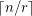Keywords:

## Directed path of length twice the minimum outdegree ★★★

Author(s): Thomassé

Conjecture   Every oriented graph with minimum outdegreecontains a directed path of length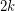.

Keywords:

## Antidirected trees in digraphs ★★

Author(s): Addario-Berry; Havet; Linhares Sales; Reed; Thomassé

An antidirected tree is an orientation of a tree in which every vertex has either indegree 0 or outdergree 0.

Conjecture   Letbe a digraph. If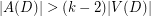, thencontains every antidirected tree of order.

Keywords:

## Decomposing an even tournament in directed paths. ★★★

Author(s): Alspach; Mason; Pullman

Conjecture   Every tournamenton an even number of vertices can be decomposed intodirected paths.

Keywords:

## Oriented trees in n-chromatic digraphs ★★★

Author(s): Burr

Conjecture   Every digraph with chromatic number at least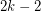contains every oriented tree of orderas a subdigraph.

Keywords:

## Discrete Logarithm Problem ★★★

Author(s):

Ifis prime and, we writeifsatisfies. The problem of finding such an integerfor a given(with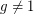) is the Discrete Log Problem.

Conjecture   There does not exist a polynomial time algorithm to solve the Discrete Log Problem.

Keywords: discrete log; NP

## Good Edge Labelings ★★

Author(s): Araújo; Cohen; Giroire; Havet

Question   What is the maximum edge density of a graph which has a good edge labeling?

We say that a graph is good-edge-labeling critical, if it has no good edge labeling, but every proper subgraph has a good edge labeling.

Conjecture   For every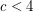, there is only a finite number of good-edge-labeling critical graphs with average degree less than.

Keywords: good edge labeling, edge labeling

## Special Primes ★

Author(s): George BALAN

Conjecture   Letbe a prime natural number. Find all primes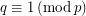, such that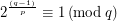.

Keywords:

## Three-chromatic (0,2)-graphs ★★

Author(s): Payan

Question   Are there any (0,2)-graphs with chromatic number exactly three?

Keywords:

## Choice Number of k-Chromatic Graphs of Bounded Order ★★

Author(s): Noel

Conjecture   Ifis a-chromatic graph on at mostvertices, then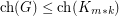.

## The Riemann Hypothesis ★★★★

Author(s): Riemann

The zeroes of the Riemann zeta function that are inside the Critical Strip (i.e. the vertical strip of the complex plane where the real part of the complex variable is in ]0;1[), are actually located on the Critical line ( the vertical line of the complex plane with real part equal to 1/2)

Keywords: Millenium Problems; zeta

## Euler-Mascheroni constant ★★★

Author(s):

Question   Is Euler-Mascheroni constant an transcendental number?

Keywords: constant; Euler; irrational; Mascheroni; rational; transcendental

## Graham's conjecture on tree reconstruction ★★

Author(s): Graham

Problem   for every graph, we letdenote the line graph of. Given thatis a tree, can we determine it from the integer sequence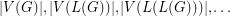?

Keywords: reconstruction; tree

## Vertex Cover Integrality Gap ★★

Author(s): Atserias

Conjecture   For every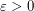there is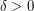such that, for every large, there are-vertex graphsandsuch that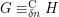and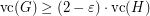.

Keywords: counting quantifiers; FMT12-LesHouches